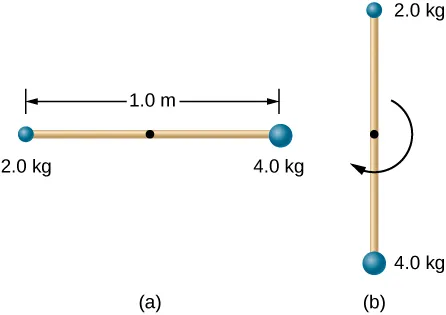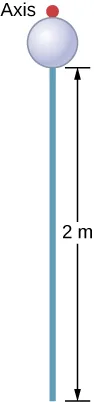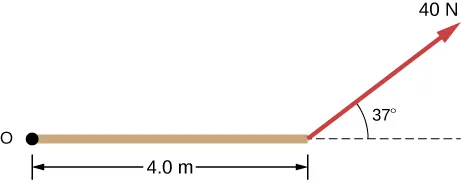University Physics Volume 1

107.

A cyclist is riding such that the wheels of the bicycle have a rotation rate of 3.0 rev/s. If the cyclist brakes such that the rotation rate of the wheels decrease at a rate of $0.3rev/s20.3rev/s2$, how long does it take for the cyclist to come to a complete stop?

108.

Calculate the angular velocity of the orbital motion of Earth around the Sun.

109.

A phonograph turntable rotating at 33 1/3 rev/min slows down and stops in 1.0 min. (a) What is the turntable’s angular acceleration, in radians/s2, assuming it is constant? (b) How many revolutions does the turntable make while stopping?

110.

With the aid of a string, a gyroscope is accelerated from rest to 32 rad/s in 0.40 s under a constant angular acceleration. (a) What is its angular acceleration in $rad/s2rad/s2$? (b) How many revolutions does it go through in the process?

111.

Suppose a piece of dust has fallen on a CD. If the spin rate of the CD is 500 rpm, and the piece of dust is 4.3 cm from the center, what is the total distance traveled by the dust in 3 minutes? (Ignore accelerations due to getting the CD rotating.)

112.

A system of point particles is rotating about a fixed axis at 4 rev/s. The particles are fixed with respect to each other. The masses and distances to the axis of the point particles are $m1=0.1kg,r1=0.2mm1=0.1kg,r1=0.2m$, $m2=0.05kg,r2=0.4mm2=0.05kg,r2=0.4m$, $m3=0.5kg,r3=0.01mm3=0.5kg,r3=0.01m$. (a) What is the moment of inertia of the system? (b) What is the rotational kinetic energy of the system?

113.

Calculate the moment of inertia of a skater given the following information. (a) The 60.0-kg skater is approximated as a cylinder that has a 0.110-m radius. (b) The skater with arms extended is approximated by a cylinder that is 52.5 kg, has a 0.110-m radius, and has two 0.900-m-long arms which are 3.75 kg each and extend straight out from the cylinder like rods rotated about their ends.

114.

A stick of length 1.0 m and mass 6.0 kg is free to rotate about a horizontal axis through the center. Small bodies of masses 4.0 and 2.0 kg are attached to its two ends (see the following figure). The stick is released from the horizontal position. What is the angular velocity of the stick when it swings through the vertical?115.

A pendulum consists of a rod of length 2 m and mass 3 kg with a solid sphere of mass 1 kg and radius 0.3 m attached at one end. The axis of rotation is as shown below. What is the angular velocity of the pendulum at its lowest point if it is released from rest at an angle of $30°?30°?$116.

Calculate the torque of the 40-N force around the axis through O and perpendicular to the plane of the page as shown below.117.

Two children push on opposite sides of a door during play. Both push horizontally and perpendicular to the door. One child pushes with a force of 17.5 N at a distance of 0.600 m from the hinges, and the second child pushes at a distance of 0.450 m. What force must the second child exert to keep the door from moving? Assume friction is negligible.

118.

The force of $20j^N20j^N$ is applied at $r→=(4.0i^−2.0j^)mr→=(4.0i^−2.0j^)m$. What is the torque of this force about the origin?

119.

An automobile engine can produce 200 N$··$ m of torque. Calculate the angular acceleration produced if 95.0% of this torque is applied to the drive shaft, axle, and rear wheels of a car, given the following information. The car is suspended so that the wheels can turn freely. Each wheel acts like a 15.0-kg disk that has a 0.180-m radius. The walls of each tire act like a 2.00-kg annular ring that has inside radius of 0.180 m and outside radius of 0.320 m. The tread of each tire acts like a 10.0-kg hoop of radius 0.330 m. The 14.0-kg axle acts like a rod that has a 2.00-cm radius. The 30.0-kg drive shaft acts like a rod that has a 3.20-cm radius.

120.

A grindstone with a mass of 50 kg and radius 0.8 m maintains a constant rotation rate of 4.0 rev/s by a motor while a knife is pressed against the edge with a force of 5.0 N. The coefficient of kinetic friction between the grindstone and the blade is 0.8. What is the power provided by the motor to keep the grindstone at the constant rotation rate?

Order a print copy

As an Amazon Associate we earn from qualifying purchases.